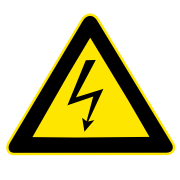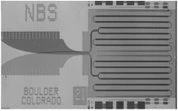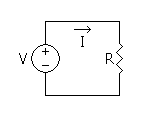## FANDOM

655 Pages

The volt (symbol: V) is the SI derived unit of electric potential difference. The number of volts is a measure of the strength of an electrical source in the sense of how much power is produced for a given current level. It is named in honor of the Italian people, physicist, Alessandro Volta  (1745–1827), who invented the voltaic pile , the first chemical battery.International danger high voltage symbol.

## DefinitionEditJosephson junction array chip developed by NIST as a standard volt.

The volt is defined as the potential difference across a conductor when a current of one ampere dissipates one watt of power. Hence, it is the base SI representation m2 · kg · s−3 · A−1, which can be equally represented as one joule of energy per coulomb of charge, J/C.

1 V = 1 W/A = 1 m2•kg•s–3•A–1

Since 1990 the volt is maintained internationally for practical measurement using the Josephson effect , where a conventional value is used for the Josephson constant , fixed by the 18th General Conference on Weights and Measures  as

K{J-90} = 0.4835979 GHz/µV.

## ExplanationEdit

The electrical potential difference can be thought of as the ability to move electrical charge through a resistance. In essence, the volt measures how much kinetic energy each electron carries. The number of electrons is measured by the charge, in coulombs. Thus the volt is multiplied by the current flow, in amperes which are one coulomb per second, to yield the total electrical power in the current, in Watts. At a time in physics when the word force was used loosely, the potential difference was named the electromotive force or emf - a term which is still used in certain contexts.

### Electrical potential difference ("voltage")Edit

Between two points in an electric field, such that exists in an electrical circuit, the potential difference is equal to the difference in their electrical potentials. This difference is proportional to the electrostatic force that tends to push electrons or other charge-carriers from one point to the other. Potential difference, electrical potential and electromotive force are measured in volts, leading to the commonly used term voltage and the symbol V (sometimes $\mathcal{E}$ is used for voltage).

Voltage is additive in the following sense: the voltage between A and C is the sum of the voltage between A and B and the voltage between B and C. Two points in an electric circuit which are connected by an ideal conductor, without resistance and without the presence of a changing magnetic field, have a potential difference of zero. But other pairs of points may also have a potential difference of zero. If two such points are connected with a conductor, no current will flow through the connection. The various voltages in a circuit can be computed using Kirchhoff's circuit laws.

Voltage is a property of an electric field, not individual electrons. An electron moving across a voltage difference experiences a net change in energy, often measured in electron-volts. This effect is analogous to a mass falling through a given height difference in a gravitational field.

### Hydraulic analogyEdit

If one thinks of an electrical circuit in analogy to water circulating in a network of pipes, driven by pumps in the absence of gravity, then the potential difference corresponds to the fluid pressure difference between two points. If there is a pressure difference between two points, then water flowing from the first point to the second will be able to do work, such as driving a turbine.

This hydraulic analogy (see separate article for full details) is a useful method of teaching a range of electrical concepts. In a hydraulic system, the work done to move water is equal to the pressure multiplied by the volume of water moved. Similarly, in an electrical circuit, the work done to move electrons or other charge-carriers is equal to 'electrical pressure' (an old term for voltage) multiplied by the quantity of electrical charge moved. Voltage is a convenient way of quantifying the ability to do work.

### Technical definitionEdit

The electrical potential difference is defined as the amount of work per charge needed to move electric charge from the second point to the first, or equivalently, the amount of work that unit charge flowing from the first point to the second can perform. The potential difference between two points a and b is the line integral of the electric field E:

$V_a - V_b = \int _a ^b \mathbf{E}\cdot d\mathbf{l} = \int _a ^b E \cos \phi dl.$

## Useful formulaeEdit

### DC circuitsEdit

$V = \sqrt{PR}$
$V = \frac{P}{I}$
$V = IR \!\$

Where V=Voltage, I=Current, R=Resistance, P=Power

### AC circuitsEdit

$V = \frac{P}{I\cos\theta}$
$V = \frac{\sqrt{PZ}}{\sqrt{\cos\theta}} \!\$
$V = \frac{IR}{\cos\theta}$

Where V=Voltage, I=Current, R=Resistance, P=True Power, Z=Impedance, θ=Phasor Angle

### AC conversionsEdit

$V_{avg} = .637V_{pk} \!\$
$V_{rms} = .707V_{pk} \!\$
$V_{rms} = .354V_{ppk}\!\$
$V_{avg} = .319V_{ppk} \!\$
$V_{avg} = .9V_{rms} \!\$
$V_{pk} = .5V_{ppk} \!\$

Where Vpk=Peak Voltage, Vppk=Peak-to-Peak Voltage, Vavg=Average Voltage, Vrms=Effective Voltage

### Total voltageEdit

Voltage sources and drops in series:

$V_T = V_1 + V_2 + V_3 + ... \!\$

Voltage sources and drops in parallel:

$V_T = V_1 = V_2 = V_3 = ... \!\$

### Voltage dropsEdit

Across a resistor (Resistor n):

$V_n = IR_n \!\$

Across a capacitor (Capacitor n):

$V_n = IX_n \!\$

Across an inductor (Inductor n):

$V_n = IX_n \!\$

Where V=Voltage, I=Current, R=Resistance, X=Reactance

## ExamplesEdit

### Voltage sourcesEditSymbolic representation of a voltage source.

Common sources of emf include:

### Common voltagesEdit1.5 volt C-cell batteries

Nominal voltages of familiar sources:

## Measuring instrumentsEditA multimeter set to measure voltage.

Instruments for measuring potential differences include the voltmeter, the potentiometer (measurement device), and the oscilloscope. The voltmeter works by measuring the current through a fixed resistor, which, according to Ohm's Law, is proportional to the potential difference across it. The potentiometer works by balancing the unknown voltage against a known voltage in a bridge circuit. The cathode-ray oscilloscope works by amplifying the potential difference and using it to deflect an electron beam from a straight path, so that the deflection of the beam is proportional to the potential difference.

## History of the voltEdit

In 1800, as the result of a professional disagreement over the galvanic response advocated by Luigi Galvani, Alessandro Volta developed the so-called Voltaic pile, a forerunner of the battery, which produced a steady electric current. Volta had determined that the most effective pair of dissimilar metals to produce electricity was zinc  and silver . In the 1880s, the International Electrical Congress, now the International Electrotechnical Commission (IEC), approved the volt for electromotive force. The volt was defined as the potential difference across a conductor when a current of one ampere dissipates one watt of power.

Prior to the development of the Josephson junction voltage standard, the volt was maintained in national laboratories using specially constructed batteries called standard cells. The United States used a design called the Weston cell  from 1905 to 1972.

## See alsoEdit

 This page uses Creative Commons Licensed content from Wikipedia (view authors).Community content is available under CC-BY-SA unless otherwise noted.